# Using quantum generative adversarial networks for portfolio analysis

For our entry into the QHack Quantum Machine Learning Hackathon our team, named QLords, decided to look into the use of quantum generative adversial networks (qGANs), QAOA and VQE for portfolio analysis.

Our code is available in this repository.

# Portfolio Analysis

In 1952, Markovitz proposed a novel theory that exploits diversification to engineer a portfolio with higher returns for lower risk, going against conventional beliefs that risk has a positive linear relationship to reward. Mean variance portfolio optimization is an analysis technique that was born from Markovitz’s work. What Markovitz added to the game was the consideration of variance and covariance between individual stocks. The idea is to maximize return by combining assets that are anti-correlated, thus resulting in a lower volatility for the portfolio.

This is not to say that we want our assets to be in direct opposition with each other (in that case we would never see any gains). We want our assets to be anti-correlated enough such that it cancels out the noise due to volatility in the short term while being positively correlated in the long term such that they both go up. The plot below shows the relationship between risk and returns. The efficient frontier is the optimized portfolio which occupies the “efficient” parts of the risk–return spectrum. This is the portfolio which satisfies the condition that no other portfolio exists with a higher expected return but with the same standard deviation of return.

# GAN Method Overview

The use of classical GANs for portfolio analysis is described in . The essence of our method is that we will end up with a model that takes in a set of data defining the stock prices of several stocks over a defined number of previous days. The model will then output it’s predicted stock data for the next period of days. We train this using a second network, a discriminator whose goal is to determine if the data (both the previous time period and the following time period) is generated or real. The generator is then trained so that the discriminator can not tell the difference between the real and fake data. These two networks are trained in turn with large quantities of data. This results in a generator that produces data that the discriminator cannot decipher if it has been generated or real. In the following diagram the training data M is split into the previous data Mb and the future data Mf. Mb can then be input into the generator, and with some noise latent vector a generated sample Mf^ can be produced. These two datasets M (Mb+Mf) and M’ (Mb+Mf^) can then be used in the training methods.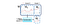Image taken from 

The data produced can then be fed into another algorithm (QAOA or VQE implementations described later) which solves the mean variance portfolio optimisation problem.

# Data

The first step is to prepare the training data. Historical stock data can be easily downloaded from the internet, in this case we have taken data from Yahoo Finance. The data is then split into sequences of the previous and future days, ready for training. The data is also normalised and converted into percentage change in order for the networks to more easily handle it. For our implementation, due to the time and computing power limitations described in more detail later, we have taken 100 sequences of TSLA data.

# Models

Ideally both the discriminator and the generator would be quantum. However the limitations on the number of qubits (32) means that compressing the data such that these two would work alongside each other would cause main features to be lost (this was tested). In our example implementation we have therefore used a classical discriminator and a quantum generator, allowing all qubits to be used as input for the generator. For the discriminator we have used a convolutional neural network as this has been shown to handle time series data well. For the generator we have used the quantum implementation below, taken from .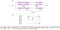Image taken from 

We have implemented this circuit with k=3, and 32 qubits, meaning there are 128 trainable parameters. We used the amplitude embedding method from PennyLane to encode the data into the intial state. For the output we take the expectation of the first 8 qubits. This means that our input data is a sequence of 32 days and it outputs the sequence for the following 8 days. Some validation data was also created in order to not overfit the model.

# Training

Due to time limitations, we were only able to run one and a half epochs for training the generator (where each epoch cycles through and trains the generator on each of the 100 sequences), and only train the discriminator once prior.

First training the classical discriminator on data produced by an initial generator that had not been trained at all. As expected this was very effective an quickly increased accuracy tending towards 100%, as shown in the tensorboard graphs below.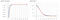Blue = Validation Data, Orange = Training Data

Training the generator was less successful, due to the limited data and epochs input. However after one epoch the generated data was significantly closer to the real validation data. In 54% of cases the model correctly predicted if the stock would increase or decrease in the next period, compared to 49% without training. This is a good accuracy for one epoch for stock prediction, in general an accuracy of 60% is widely accepted to be a good model.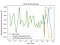Result Sample After 1 Epoch with limited data

# Devices

To run these models we used an array of simulators and devices:

1. FLOQ — Having won access to Google’s in development quantum simulator it became incredibly useful in our training process. The simulator significantly sped up the process of creating the code and debugging it so that time on the real devices was best spent. FLOQ is optimised for 32 qubits which is ideal for our circuit, and sped up the circuit run time from O(500 seconds) on SV1 to O(50 seconds). This allowed our preliminary testing to be as quick as possible.
2. SV1 — Amazon Braket’s SV1 simulator proved useful in testing the optimisation of the circuit parameters, as the parallel feature meant that multiple circuits could run in parallel, this was used to finalise our testing of the model.
3. Rigetti — Having won the \$4000 AWS credits power up, we could run our final model through Amazon Braket on the Rigetti machine.

# Limitations

For this project we had the following limitations imposed upon us:

1. The devices available imposed a maximum of 32 qubits.
2. The run time of the training could not be longer than the period Rigetti was open for.

This meant a few compromises were made, that would be changed given bigger computers or more time. The following method would be used in the ideal case:

All of the 22350 sequence datasets produced would be used in the training of the model, as modelling time series data takes a notoriously large amount of data to become effective. Furthermore each sequence could be expanded to include more data and other indicators such as volume. Then, when implementing the models, the generator would take in the data for all assets at once and output the predictions for all of the assets. If the datasets are larger a quantum CNN could also be implemented on the large quantity of data, as it is in the classical implementation of GANs for time series as described in . Large datasets would be needed for this in order to not lose any key information in the convolutions. Finally the discriminator would also be quantum and can be connected to the same output wires of the generator, meaning that the GAN is entirely quantum rather than partially.

If this model was implemented with more iterations of training the generator and discriminator alternately it would extrapolate the small increase we saw in our implementation and produce a much better model that can then be used for portfolio analysis.

# Using the Model for Portfolio Analysis

As the data our model produced is not complete, for the portfolio analysis implementation using QAOA we take raw historical data to compare the quantum methods against classical benchmarks. If we had the model described above the data used in this would be replaced with the predictions made by the generator, allowing for future prices to be accounted for in the portfolio model. Furthermore the accuracy of the predictions can be included and a combination of past data and future predictions, weighted according to this risk of the predictions being incorrect can be incorporated into solving the portfolio optimisation problem.

# Mathematical Description of Portfolio Analysis

The mean-variance portfolio optimization problem is a NP-Hard COP, making it computationally expensive for current classical computers to solve. Mathematically, we can represent this quadratic problem for n assets as: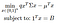Mathematical Description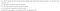Definitions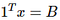Equality Constraint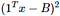Penalty Term

For our implementation, we simplified the conditions such that all of the budget must be spent and normalized all equity values to 1.

Our equality constraint states that the number of ones in our binary decision variable vector must be equal to the budget (we spend everything). We create a penalty term based upon this constraint which is scaled by a parameter and subtracted from the objective function.

# QAOA and VQE Implementations

The problem described in the previous section can be mapped into a Hamiltonian whose ground state corresponds to the optimal solution for the mean-variance portfolio problem. An implemetation of the Variational Quantum Eigensolver (VQE) and the Quantum Approximate Optimization Algorithm (QAOA) can then be used to find the optimal solution for a given set of equities . Due to time constraints we took some pre defined functions from Qiskit Aqua  and used them to implement the solution in PennyLane. In our implementation we have also further backtested each approach against three random stock portfoliios from the S&P 500. The results of this are shown below (showing the growth of the portfolios over time).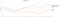Trial 1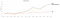Trial 2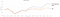Trial 3

As we can see on these portfolio trials the QAOA implementation performs on average better than the VQE, however not quite as well as the classical Eigensolver with current models. However we can see that the methods clearly work, and when bigger quantum computers are available this will be one of the problems that has the potential to have a big impact on the financial industry. Furthermore if the constraints are improved to be more general, even more improvement could arise.

# Tensor Networks

There are some further methods that had we had more time we could implement to improve our model. The methods described in  can be applied to the qGAN. These improvements used a tensor network based generative model. As tensor networks are adaptive, dimensions of tensor indices internal to the network grow and shrink during training to concentrate resources on the particular correlations within the data. In our case these are extremely important as we’re talking about different portfolios. The workflow planned for this was constructing the MPS first and then optimising it through a DMRG algorithm. All initial cost function evaluations were to be decided by our qGAN, and is constructed just to give support to the target probability distribution the MPS model was aiming to capture. MPS would have significantly decreased the time complexity of calculating the cost function.